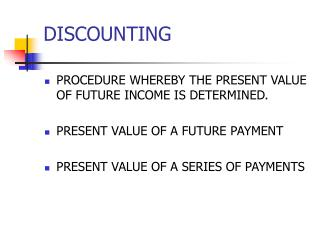DownloadDownload PresentationDISCOUNTING

# DISCOUNTING

Télécharger la présentation## DISCOUNTING

- - - - - - - - - - - - - - - - - - - - - - - - - - - E N D - - - - - - - - - - - - - - - - - - - - - - - - - - -
##### Presentation Transcript

1. DISCOUNTING • PROCEDURE WHEREBY THE PRESENT VALUE OF FUTURE INCOME IS DETERMINED. • PRESENT VALUE OF A FUTURE PAYMENT • PRESENT VALUE OF A SERIES OF PAYMENTS

2. PRESENT VALUE OF A FUTURE PAYMENT PVO = FVN /(1+i)n OR PRESENT VALUE = FUTURE VALUE / (1 + COMPOUND RATE) CONVERSION PERIODS

3. Present Value Single Deposit (Graphic) Assume that you need \$5,000in 2 years. Let’s examine the process to determine how much you need to deposit today at a discount rate of 7%. 0 12 7% \$5,000 PV0 PV1

4. Present Value Single Deposit (Formula) PV0 = FV2 / (1+i)2 = \$5,000/ (1.07)2 = FV2 / (1+i)2 = \$4367.19 0 12 7% \$5,000 PV0

5. General Present Value Formula PV0= FV1 / (1+i)1 PV0 = FV2 / (1+i)2 General Present Value Formula: PV0 = FVn / (1+i)n or PV0 = FVn (PVD i,n) -- See Table A2 etc.

6. Valuation Using Table A2 PVD i,nis found on Table A2

7. Using Present Value Tables PV2 = \$5,000 (PVD 7%,2) = \$5,000 (.873) = \$4365.00

8. PROBLEM: \$6298.56 DISCOUNTED @ 8% FOR 3 YEARS PVO = 6298.56/(1.08)3 PVO = 6298.56/(1.259712) PVO = 5000

9. Example Problem Julie Miller wants to know how large of a deposit to make so that the money will grow to \$10,000in 5 years at a discount rate of 10%. 0 1 2 3 4 5 10% \$10,000 PV0

10. Problem Solution • Calculation based on general formula: PV0 = FVn / (1+i)nPV0= \$10,000/ (1+ 0.10)5 = \$6,209.21 • Calculation based on Table A2: PV0= \$10,000(PVD 10%, 5)= \$10,000(.6209) = \$6,209.00